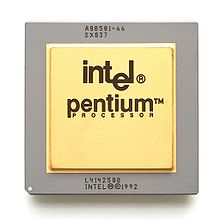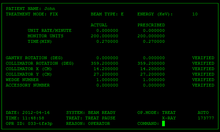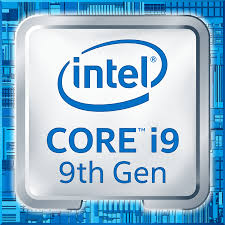## Automating Mathematics?

Department of Mathematics

Indian Institute of Science

Bangalore

• Can computers acquire all the major capabilities used by mathematicians and the mathematics community in the discovery and proof of mathematical results and concepts?
• When? How?
• Computers are already used in several ways.
• Capabilities in other cognitive domains that suggests they can do much more.
• Automated theorem proving is closely related to computer verification of proofs.

# Mathematics

Cdang Derivative work: Muskid, CC BY-SA 3.0, via Wikimedia Commons

### Universal deducer?

• A universal deducer is a program which, given a mathematical statement, either proves it is true or proves it is false.
• By results of Church, Gödel, Turing, such a program is impossible.
• Practically, we can conclude that there is no best deducer, as any given proof can be found by some deducer but no deducer can find all proofs.

### Some computer-assisted proofs

• Four-colour problem: Any map can be coloured with at most $4$ colours.
• Kepler Conjecture: The most efficient way to pack spheres is the hexagonal close packing.
• Boolean Pythagorean triples problem: Is it possible to colour the positive integers either red or blue, so that if three integers $a$, $b$, $c$, satisfy $a^{2}+b^{2}=c^{2}$, they are not all the same colour?
• Smale conjecture for hyperbolic $3$-manifolds.

### Some ways computers are used

• Numerical computation.
• Enumeration.
• Symbolic and computational algebra.
• Compact enumeration.
• Exact real number arithmetic.
• Linear programming.
• Decidability of real semi-algebraic geometry.
• SAT solvers and SMT solvers.

### Robbins conjecture

• Robbins conjecture was a conjectural characterization of Boolean algebras in terms of associativity and commutativity of $\vee$ and the Robbins equation $\neg(\neg(a\vee b)\vee \neg(a \vee \neg b)) = a$.
• This was conjectured in the 1930s, and finally proved in 1996 using the automated theorem prover EQP.
• So far, this seems to be the only major success of fully autonomous deductive theorem provers.

# Interactive Theorem Provers

### Interactive Theorem Provers

• Interactive Theorem Provers are software systems where proofs are obtained by human-machine collaboration.
• The computer both finds (parts of) proofs and verifies correctness.
• The ease of proving in such systems depends on how concise and composable proofs are and the strength of its elaborator and tactics.
• The former depends on foundations and the latter is essentially automated theorem proving.

### Who guards the guards?

• A computer verified proof is only as trustworthy as the system that verified the proof.
• Following the de Bruijn principle, proofs are verified by a small trusted kernel, which can be thoroughly checked.
• For example, the lean theorem prover has three (small) proof checkers written in three languages.
• The Feit-Thompson theorem has been formalized in the system Coq by Georges Gonthier and others.
• The lean mathematical library has formalizations of a lot of undergraduate mathematics and many advanced results.
• These not only show that formalization is feasible, but can form data for both machine learning and advanced semantic searches and other tooling.

# Formal methods

## Mathematical proofs elsewhere

### Formal methods

• We specify (describe) software, hardware etc. in precise mathematical terms.
• We give mathematical proofs of correct behavior, which are computer verified.
• This gives a much greater certainty of correctness.
• However, proofs are much harder than tests.
• Formal proofs use interactive theorem provers; with better provers we can prove more often.

#### Do we need completely correct always?Pentium FDIV Bug Fixing an error is very costlyTherac 25 radiation machine Safety criticalWhatsApp Pegasus attack A bug is a vulnerability

#### Some users of formal methodsIntel Chips Fixing an error is very costlyParis driverless metro Safety criticalScala dotty compiler A bug is a vulnerability

# and Games

#### Programming a Computer for Playing Chess

• Playing Chess can be based on
• judging the value of a fixed players position.
• a policy: sequences of moves to consider.
• We compute (and use) the value at the end of sequences moves we consider.
• We recursively decide the best moves by minimax — alternately maximizing and minimizing.
• We refine using various heuristics, such as quiescence search and $\alpha-\beta$ pruning.
• Openings give a policy, as do endgame tables.

### Kasparov vs Deep Blue

• In 1997, a computer Deep Blue defeated the Chess world champion Gary Kasparov.
• Deep Blue was based on extending the above methods to elaborate (rule based) values and policies (chess theory), and improved heuristics.
• However, Deep Blues was very limited in certain capabilities.
• The value and policy functions of Kasparov were far better, but compensated for by Deep Blue being able to consider far more move sequences.

### AlphaGo vs Lee Sedol

• In the chinese game Go, the number of legal moves is much larger, so trying everything means we cannot look many moves ahead.
• More importantly, it is very hard to describe a good value function (we use tacit knowledge).
• This makes it far harder for computers.
• Yet, in March 2016, a Go playing system AlphaGo defeated 18-time world champion Lee Sedol.
• In January 2017, AlphaGo defeated the world number one Ke Jie comprehensively.

### AlphaGo and Learning

• The policy and value functions of AlphaGo are deep neural networks that were trained.
• The policy network was initially trained by learning to predict the next move from games of expert players (behaviour cloning).
• The value network was trained by AlphaGo playing against versions of itself.
• AlphaGo considered fewer sequences of moves than Deep Blue.
• AlphaGo came up with unexpected moves.

### Deep learning (policy).

• We search for an optimal policy in a space of functions smoothly parametrized by $\mathbb{R}^N$ with respect to an appropriate smooth loss.
• The space of functions we consider are compositions of the form $$\Phi = \Psi_k\circ \Psi_{k -1}\circ \dots \circ \Psi_1,$$ where the functions $\Psi_j$ are layers, and are smoothly parametrized by $\mathbb{R}^{n_j}$, with $N = n_1 + n_2 + \dots + n_j$.
• A dense layer is a function of the form $y = \sigma(Ax + b)$, where $A= (a_{ij})$ is a linear function, $b$ a vector, and $\sigma$ is $x\mapsto e^x/(1 + e^x)$ applied component-wise.
• This is parametrized by entries of $A$ and $b$.
• In a convolutional layer, the linear function $A$ is a convolution, e.g., $y_j = \sum_{k} a _k x_{j - k}$.
• To get a probability distribution, we use as the last layer softmax: $y_j = \frac{e^{x_j}}{\sum_i e^{x_i}}$.
• Optimization is by variations of gradient flow.
• By chain rule, this can be computed layer-by-layer (backward propagation).

### AlphaGo Zero and Alpha Zero

• AlphaGo was succeeded (and defeated) by AlphaGo Zero, which learnt purely by self play.
• Its successor, AlphaZero, could master a variety of similar games starting with just the rules.
• AlphaZero took just 4 hours to become the strongest chess player on the planet (beating a traditional chess program, Stockfish).
• AlphaZero “had a dynamic, open style”, and “prioritizes piece activity over material, preferring positions that looked risky and aggressive.”

# Artificial Intelligence elsewhere

### Word Embeddings

• To give words a structure and capture relations, words are embedded as points in space.
• To do this, (in Word2Vec) we set up the problem of predicting a word given its neighbours.
• We look for solutions of this problem that involve mapping words into space, and predicting from neighbours using the points.
• Analogies such as Paris is to France as Madrid is to Spain are captured by vector operations.

### Generative Query network

• In an artificial 3D environment, the network observes 2D images from a few positions.
• It has to predict the observed image from a new position.
• To do this, the 2D image was mapped to a concise representation by a network, which was then used to predict the image from a different viewpoint.
• The concise representation factorized by colour, shape and size (among other things).

• These consist of a pair of networks, contesting with each other.
• One network generates candidates (generative) and the other evaluates them (discriminative).
• For example the discriminative network tries to distinguish between real images and synthetic ones generated by the generative network.

### Distributional reinforcement learning

• In temporal reinforcement learning, a network tries to predict (average) future rewards.
• However, sometimes the reward is either very big or very small, so the average reward is misleading.
• In distributional reinforcement learning we have several predictors, which react differently to positive and negative errors.
• Recently, similar distributions of dopamine cells was found in the brains of mice.

### Attention is all you need

• The meaning of a word depends on the context, i.e., other words surrounding it.
• In the transformer architecture, this is captured by learning to which other words to pay attention for determining the next representation.
• The encoding of words includes position vectors, defined by using harmonics.
• This has lead to revolutionary improvements, including forming the basis for GPT 3.
• Attention networks are used in AlphaFold 2.

### Emergent Semantics?

• In image processing, successive layers capture features at increasing levels of abstraction.
• Often (parts of) networks are shared, to better capture structure and meaning. For example, AlphaGo Zero learnt better in part by having a single neural network for policy and value.
• In zero-shot translation, a network translated between new pairs of languages; apparently based on an internal language.

#### Capabilities of Artificial Intelligence

• Make moves that we can appreciate.
• Judge value based on future rewards.
• Show originality.
• Acquire tacit (to us) knowledge.
• Work with limited and/or unstructured data, by self-play, using synthetic tasks and by self-supervised learning.
• Organize objects naturally and efficiently, capturing composition, abstraction etc.
• Discover concepts by solving problems?

# Mathematical Proofs?

• We can iteratively do and improve at doing:
• accumulate knowledge.
• digest the knowledge.
• solve (& pose) problems, prove theorems:
• to accumulate knowledge,
• as exercises to digest knowledge.
• develop judgements: truth, importance, difficulty.
• Results may stagnate or be confined to some types and/or some areas.
• We need good constructions and choices for:
• outcomes,
• composite moves, hence parameters for policy,
• integration with experimentation,
• integration with domain specific solvers and other software,
• integration with (human) mathematics,
• strategies: e.g., how long to keep trying.
• Machine learning is being used in Automated and Interactive theorem proving for premise selection as well as choosing tactics and parameters.
• I have been working on a system which I call ProvingGround along the lines sketched here.
• It is also valuable to be able to learn from existing mathematics, which could use formalized libraries and natural language processing.
• However, for computer proofs to be useful, we would like to learn from computer proofs.
• A question on a blog of Terence Tao, asked to him by Apoorva Khare, was answered in PolyMath 14.
• A crucial step in the discovery was a computer generated but human readable proof I posted.
• The computer proof was studied by Pace Nielsen, who extracted the internal repetition trick.
• This was extended by Pace Nielsen and Tobias Fritz and abstracted by Terence Tao.
• From this Fritz obtained a key lemma, which was used by Terrence Tao to answer the question in the negative, and give a more general result.
• This was published as PolyMath 14 with 6 authors (including Lior Silberman).
• A natural question is the replicability of the computer proof, which was originally produced in an interactive session.

Indeed, a script gives a similar proof.